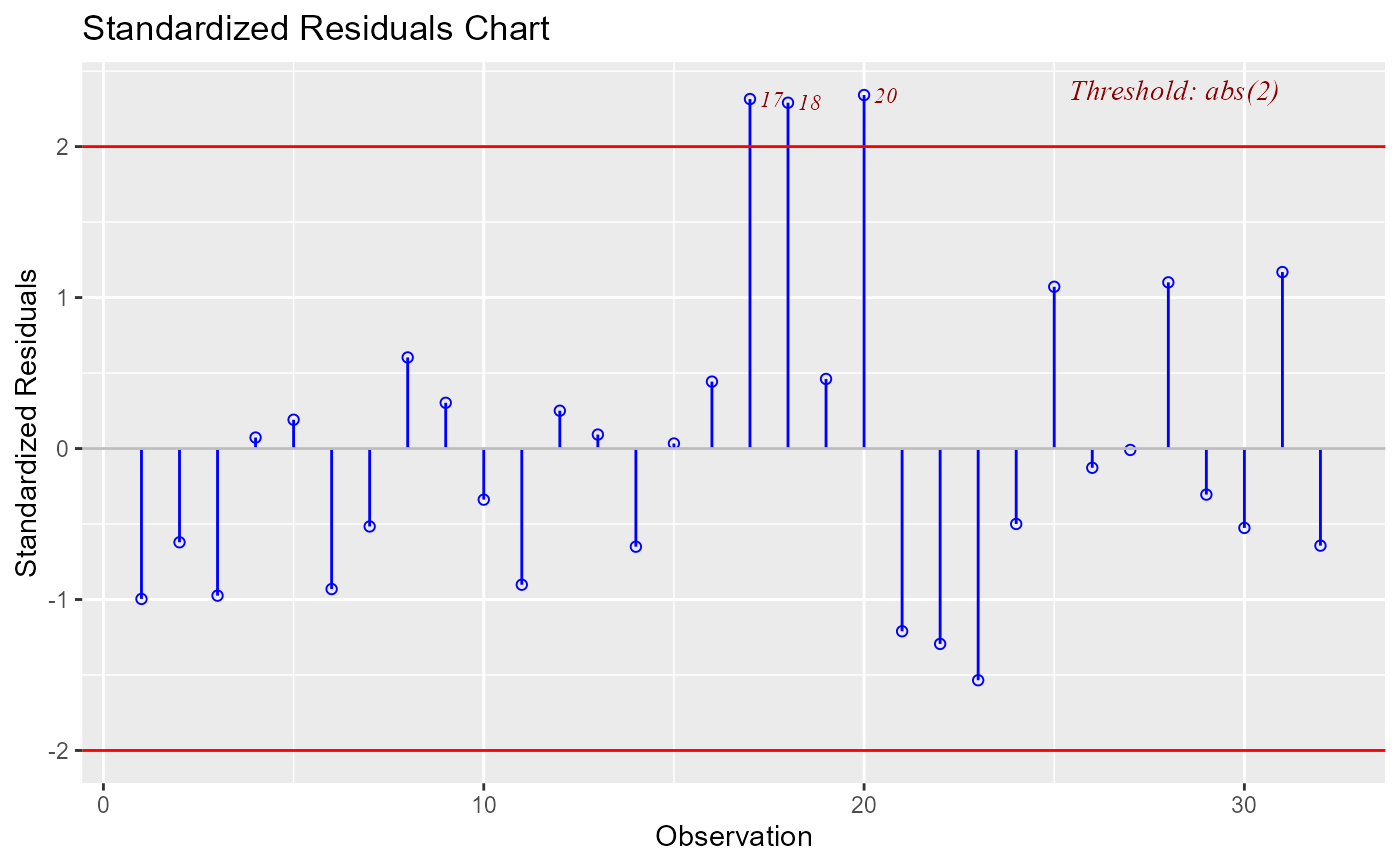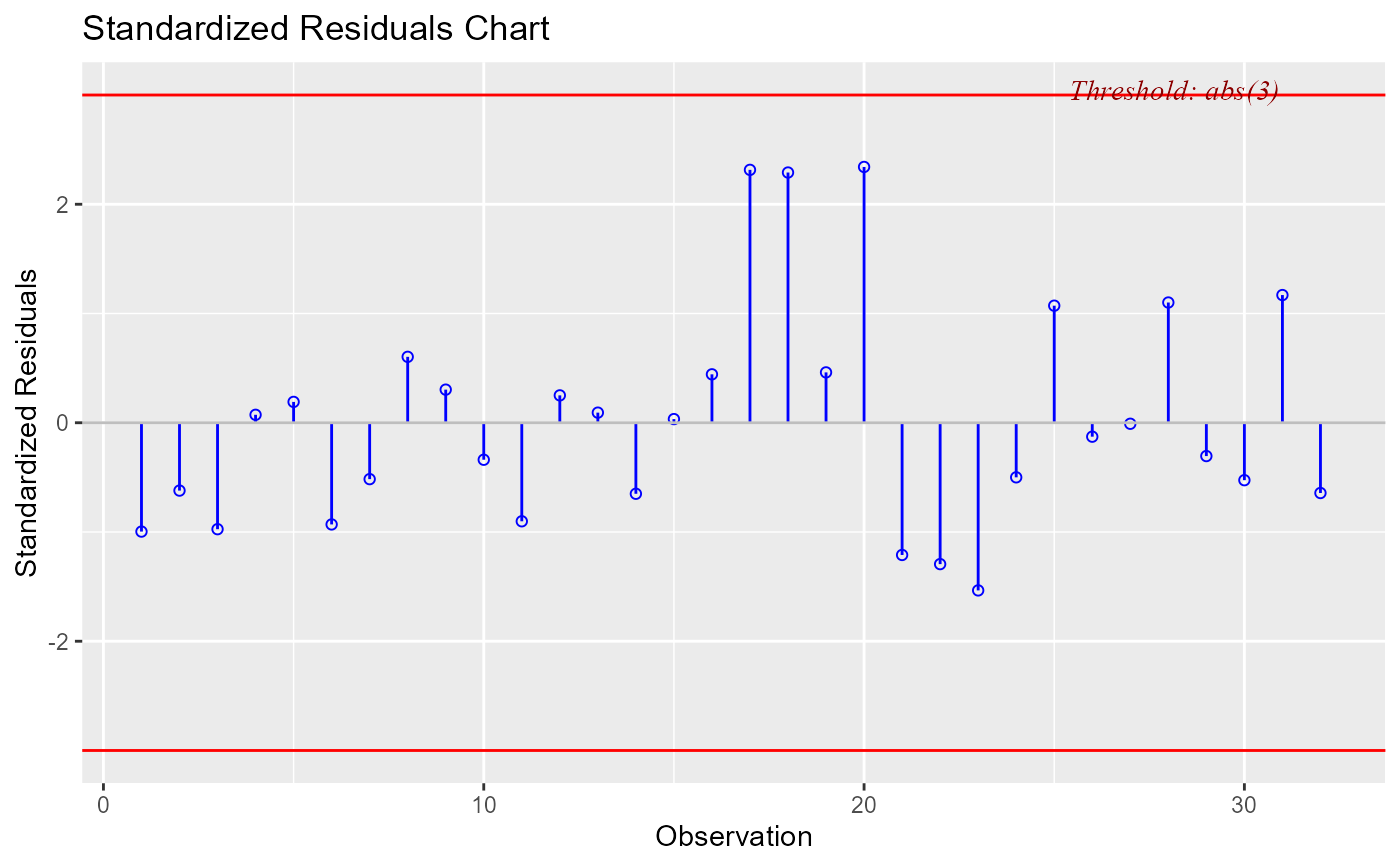Chart for identifying outliers.

ols_plot_resid_stand(model, threshold = NULL, print_plot = TRUE)

## Arguments

model An object of class lm. Threshold for detecting outliers. Default is 2. logical; if TRUE, prints the plot else returns a plot object.

## Value

ols_plot_resid_stand returns a list containing the following components:

outliers

a data.frame with observation number and standardized resiudals that exceed threshold

for classifying an observation as an outlier
threshold

threshold for classifying an observation as an outlier

## Details

Standardized residual (internally studentized) is the residual divided by estimated standard deviation.

## Deprecated Function

ols_srsd_chart() has been deprecated. Instead use ols_plot_resid_stand().

model <- lm(mpg ~ disp + hp + wt, data = mtcars)ols_plot_resid_stand(model, threshold = 3)